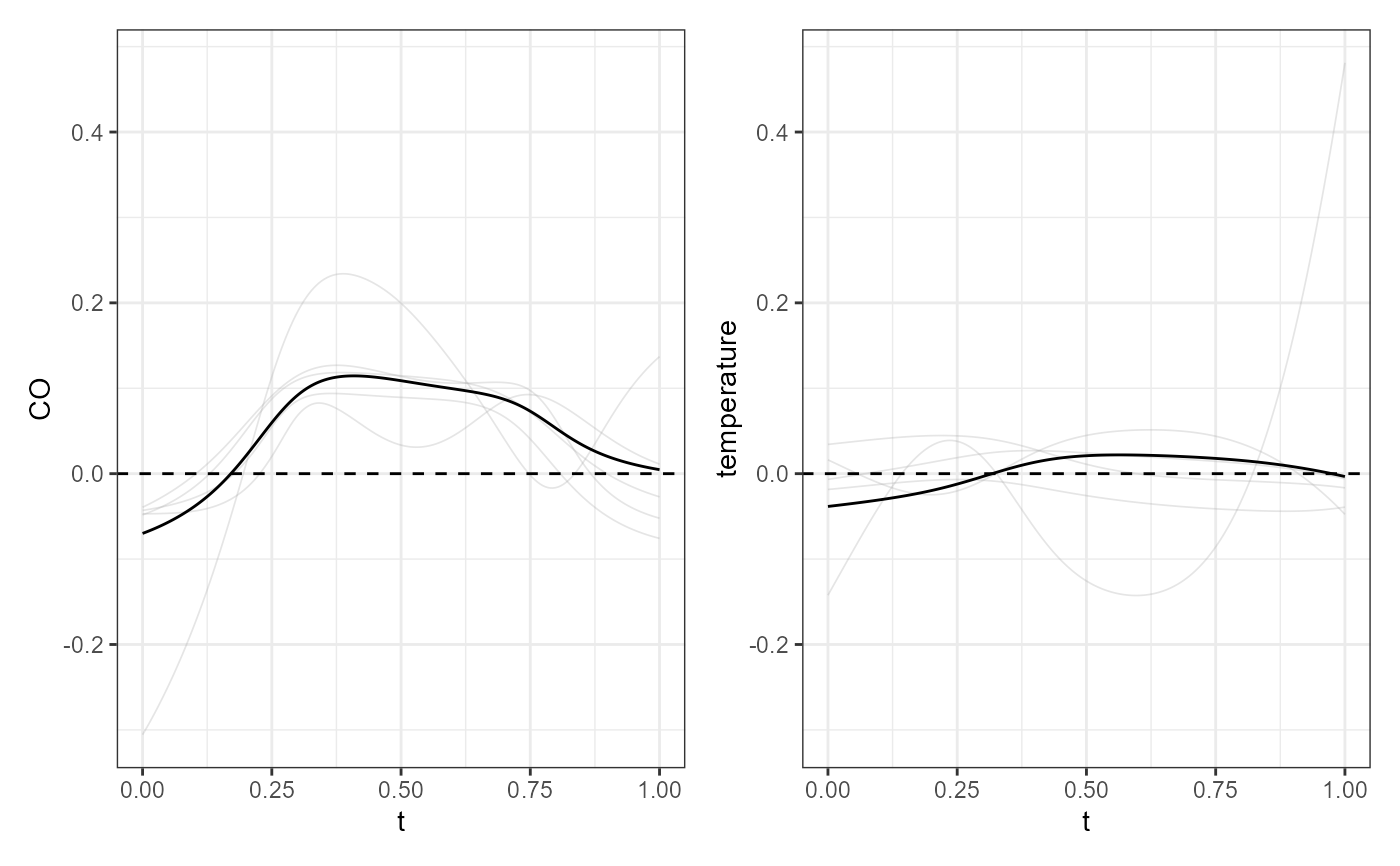Plot bootstrapped estimates of the scalar-on-function regression coefficient for empirical uncertainty quantification. For each iteration, a data set is sampled with replacement from the training data use to fit the model, and the regression coefficient is estimated.

plot_bootstrap_sof_pc(mod, nboot = 25, ncores = 1)

## Arguments

mod

A list obtained as output from sof_pc, i.e. a fitted scalar-on-function linear regression model.

nboot

Number of bootstrap replicates

ncores

If you want estimate the bootstrap replicates in parallel, give the number of cores/threads.

## Value

A ggplot showing several bootstrap replicates of the multivariate functional coefficients estimated fitting the scalar-on-function linear model. Gray lines indicate the different bootstrap estimates, the black line indicate the estimate on the entire dataset.

## Examples

library(funcharts)
data("air")
air <- lapply(air, function(x) x[1:10, , drop = FALSE])
fun_covariates <- c("CO", "temperature")
mfdobj_x <- get_mfd_list(air[fun_covariates], lambda = 1e-2)
y <- rowMeans(air\$NO2)
mod <- sof_pc(y, mfdobj_x)
plot_bootstrap_sof_pc(mod, nboot = 5)